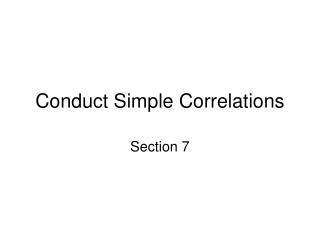DownloadDownload PresentationConduct Simple Correlations

# Conduct Simple Correlations

Download Presentation## Conduct Simple Correlations

- - - - - - - - - - - - - - - - - - - - - - - - - - - E N D - - - - - - - - - - - - - - - - - - - - - - - - - - -
##### Presentation Transcript

1. Conduct Simple Correlations Section 7

2. Correlation • A Pearson correlation analyzes relationships between parametric, linear (interval or ratio which are Scale in SPSS) variables. If ordinal, use Spearman Rho even if not from a normal distribution. • You can enter several variables and get a matrix of the direction and strength (-1 to 1) of relationships.

3. To examine the relationship between GPA and Confidence, start by restating the hypothesis. • Hypothesis: • It is hypothesized that there will be a significant positive relationship between GPA and Level of Confidence . • This is directional so it is one-tailed. • Variables and Level of Measurement: • Variable1: GPA (Scale) • Variable 2: Level of Confidence (Scale)

4. Select: Analyze > Correlate > Bivariate • Highlight GPA and the Confidence questionon the list and click on the arrow to move them to the Variables box. • Check Pearson, One-tailed, and Flag significant correlations. • Select: Options. Check Means and standard deviations. Select: Continue > OK

5. Review Output • Descriptive Statistics: Here are the Means, Standard Deviations, and N for GPA and Level of Confidence. • Correlations: The variables are listed across the top and down the side so that they intersect within the grid. Each intersection box has the value of the correlation, then the significance level, then the N.

6. Find the numbers in the statement below on the output at the intersection of GPA and Level of Confidence (the question about confidence): • There was a positive correlation [r(10)= .883, p < .01] between GPA and Level of Confidence.

7. Scatter plot • A scatter plot is a common method of displaying the results of a bivariate correlation. You can add a third variable by entering it at set markers by. • One variable is represented on each axis and the dots represent the intersection of participants’ scores on the two variables.

8. Select: Graphs > Scatter/Dot > Simple Scatter > Define • Highlight GPA. Click the arrow to move it to the Y Axis box. • Highlight Level of Confidence question. Click the arrow to move it to the X Axis box.

9. Select: Titles. • In the Footnote Line 1 box, type “Figure 1. There was a significant positive relationship between GPA and Level of Confidence.” • Select: Continue > OK

10. SPSS Graphs are easily edited and copy/pasted into your document. You can change the Axis labels, colors, sizes, etc. in the Chart Editor. • Place your curser over the chart and double-left click. Now double click on the X Axis label (On a scale of 1 to 10 with 10 being the most, how confident are you that you will learn statistics?). A the blue box appears around the text. (Close the Properties box that pops up.) • You can now change the label by deleting the old label and typing: “Level of Confidence”

11. Click outside the box onto the SPSS Viewer to close the Chart Editor and the change will be made to your graph. • If you wanted to use your graph in a document, you would just right click then Copy / Paste it into your document.

12. Good Job! • You have almost completed the module. • Just 2 more steps.